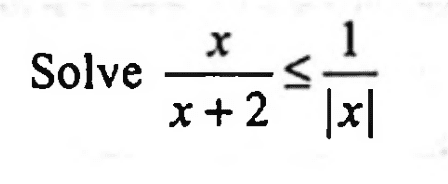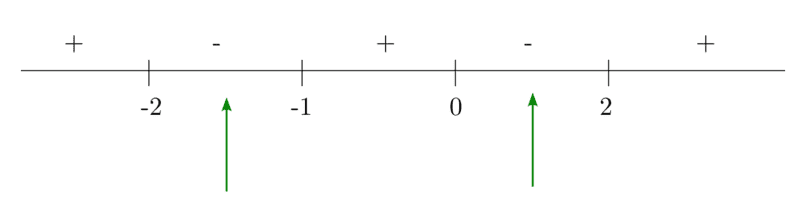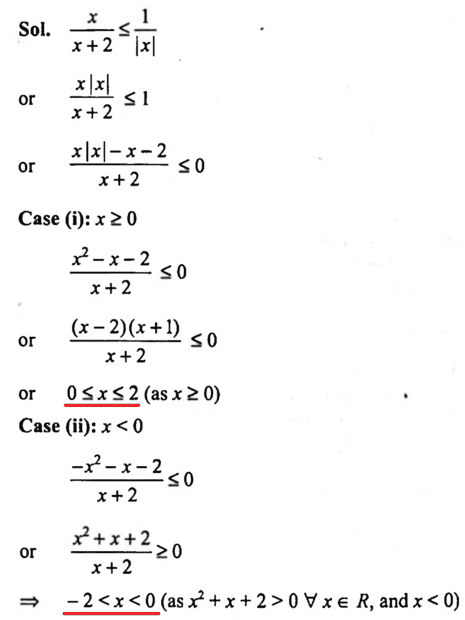# Solving for ##x## for a given inequality

• brotherbobby

#### brotherbobby

Homework Statement
Solve : ##\mathbf{\dfrac{x}{x+2}\le \dfrac{1}{|x|}}##
Relevant Equations
(1) By definition, ##|x| = x## if ##x\ge 0## and ##|x| = -x## if ##x \le 0##.
(2) ##\frac{1}{x}## is not defined for ##x = 0##.
(3) If ##|x| > a\Rightarrow x>a\; \text{or}\; x<-a##Problem Statement :
I copy and paste the problem from the text to the right.

Attempt (mine) : Given the inequality ##\dfrac{x}{x+2}\le \dfrac{1}{|x|}##. We see immediately that ##x\ne 0, -2##. At the same time, since ##|x|\ge 0\Rightarrow \frac{x}{x+2}\ge 0##.

Now if ##\frac{x}{x+2}\le \frac{1}{|x|}\Rightarrow \frac{1}{|x|}\ge \frac{x}{x+2}##, then we have either ##\frac{1}{x}\ge \frac{x}{x+2}## or ##\frac{1}{x}\le -\frac{x}{x+2}##.

1. The first inequality above reduces to ##\frac{1}{x}-\frac{x}{x+2}\ge 0\Rightarrow \frac{x+2-x^2}{x(x+2)}\ge 0\Rightarrow \frac{x^2-x-2}{x(x+2)}\le 0\Rightarrow \frac{(x-2)(x+1)}{x(x+2)}\le 0## which lead to the answers : ##\boxed{-2<x<-1\; \text{OR}\; 0<x<2}## (note the equality condition is forbidden due to considerations right at the beginning). This can be found by the number linepneumonic shown to the right or any other way you are used to with inequalities.

I attach the pictorial pneumonic to the right for those who are used to it.

2. The second inquality above reduces to ##\frac{1}{x}+\frac{x}{x+2}\le 0\Rightarrow \frac{x^2+x+2}{x(x+2}\le 0##. Now we can show using the method of "squaring a quadratic equation" that the numerator ##x^2+x+2>0 \;\;\forall x##. Thus focus falls on the numerator, which yields ##\boxed{-2<x<0}##.

Putting both cases 1 and 2 together and caring for overlaps, my solution for the proble reads : ##\boxed{-2<x<-1\;\;\;\text{OR}\;\;\; 0<x<2}## .

Solution (Text) : I could neither follow, nor agree, with the solution in the text. I copy and paste it below.I have underlined what I believe to be the solutions. So ##x=0?!##. Clearly that is not correct. However, the text is right when I take samples from its solutions that are less than zero, for instance ##x = -1, -0.5## etc., solutions which I did not get. Surely my method is mistaken.

A hint or suggestion would be welcome.

Your case 1 is when ##x>0## thus the solution ##-2<x<-1## must be disregarded as out of the allowed limit (which is ##x>0##).

•FactChecker
In addition to @Delta2's point, you have combined your two 'ORed' cases incorrectly. You should have included all of the -2<x<0 results of the second case.

I attach the pictorial pneumonic
"Pneumonic" isn't the word you want here. That would be "mnemonic."

••nuuskur and Delta2